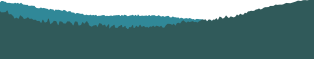PyCon 2016 in Portland, Or## Kalman Filters for non-rocket science

Audience level:
Intermediate
Category:
Other

### Description

Kalman Filters have been widely used for scientific applications. No wonder people often think they involve complex math, however you can actually introduce the Kalman Filter in your daily data processing work, without the complex math you would imagine. This talk will show how to implement the discrete Kalman Filter in Python using NumPy and SciPy.

### Abstract

A Kalman Filter is a mathematical algorithm that provides an efficient way to calculate recursively the state of a system when new measurements are entered without recalculating everything all over again, minimizing the propagation of errors in measurements and state calculation. The Kalman Filter is very powerful and can provide you estimations of past, present and future states of your system. The most common application for Kalman Filters are in navigation and trajectory calculation for aircrafts and spacecrafts. NASA has implemented Kalman Filters for Apollo 13 and 14 Navigation, in Assembly Language (https://github.com/eramirem/virtualagc/blob/1474cb493d8b6e30cc8142a2d7d8a135aea4b051/Luminary131/KALMAN_FILTER.agc) The framework for the Kalman Filter is a system is represented by a collection of equations like: Au = B Being A the coefficient matrix, u the state of the system, and B the measurements. Everytime a new measure comes, we must update the state of the system, using an equation that describe the change of the state due to the incoming measure: u₁ = F₀u₀ + epsilon₀ This describes the change of state between time 0 and time 1; being F₀ a function applied on u₀ and epsilon₀ some errors on the state equation. The Kalman Filter is basically a predict-update algorithm. It uses new information to get a better estimate of the state. If you think about it, a discrete system can be analogous to a time series: At a discrete time a measure comes in and the state of the system is updated (being the state any function over your data), so it is perfectly fine to think of the Kalman Filter as an online algorithm for segmenting time series. SciPy provides all the core Linear Algebra we need for predictions and updates and NumPy provides the data structures to hold the equations of state.